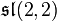# Nilpotent Lie rings of order 8

This article gives information about, and links to more details on, algebraic structures of the type nilpotent Lie ring whose order (i.e., the size of the underlying set) is 8.
See pages on algebraic structures of order 8|See pages on nilpotent Lie rings of a particular order

## Statistics at a glance

Quantity Value
Total number of nilpotent Lie rings 5
Number of abelian Lie rings 3
Number of non-abelian Lie rings of class two 2
To understand these in a broader context, refer nilpotent Lie rings of order 2^n|nilpotent Lie rings of prime-cube order

## The list

Common name for Lie ring Nilpotency class Isomorphism class of additive group Generic name for arbitrary prime
cyclic Lie ring of order 8, additive group cyclic group:Z8 1 cyclic group:Z8 abelian Lie ring, additive group cyclic group of prime-cube order
abelian Lie ring of order 8, additive group direct product of Z4 and Z2 1 direct product of Z4 and Z2 abelian Lie ring, additive group direct product of cyclic group of prime-square order and cyclic group of prime order
abelian Lie ring of order 8, additive group elementary abelian group:E8 1 elementary abelian group:E8 abelian Lie ring, additive group elementary abelian group of prime-cube order
niltriangular matrix Lie ring:NT(3,2) -- also isomorphic to the special linear Lie ring$\mathfrak{s}\mathfrak{l}(2,2)$ 2 elementary abelian group:E8 niltriangular matrix Lie ring:NT(3,p). Note that in characteristics other than 2, the special linear Lie ring$sl(2,p)$ is not isomorphic to$NT(3,p)$. In fact, it is not even a nilpotent Lie ring and is instead a simple Lie ring.
semidirect product of Z4 and Z2 as Lie rings 2 direct product of Z4 and Z2 semidirect product of cyclic Lie ring of prime-square order and cyclic Lie ring of prime order